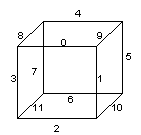## Class SoEdgeFlag

• All Implemented Interfaces:
`SafeDisposable`

```public class SoEdgeFlag
extends SoNode```
Class to mark/unmark edges for beveling. This node defines the current marked/unmarked edges for all subsequent shapes.

The marking/unmarking of the edges is specified using an int_32t array. The meaning of this array and its elements depends on the shape it is applied to.

• For an `SoIndexedFaceSet`, each element of the array is an index in the `SoIndexedShape.coordIndex` field. The vertex corresponding to this index is the first vertex of the edge. The other vertex of the edge is the next vertex in the face according to the order of the vertices in the `SoIndexedFaceSet` array. If the next element in the array is -1 (end of face), the next vertex is the first vertex of the current face (as for edge (5,3) in the example below). Otherwise, the next vertex is the next element in the array (as for edge (1,2) in the example below). The same edge can appear in two different faces, but it is not necessary to mark it in both faces; only one mark per edge is needed.

Example:

 0 1 2 3 4 5 6 7 8 9 10 11 coordIndex = [ 0, 1 , 2 , -1, 3 , 4, 5 , -1, 2, 1, 3, -1 ] edgeMarks = [ 1 => edge (1, 2), 6 => edge (5, 3) ]

`SoFaceSet`
• For an `SoFaceSet`, the marking is a little different from the `SoIndexedFaceSet`. Each element is an index directly in the coordinate array. This corresponds to the first vertex of the edge; the other is the next vertex in the same face. As each face description in the coordinate array is cyclic, the other vertex is either the next vertex in the array, or the first vertex of the face if it is the end of the face.

Example:

 coordinates = [{0.0, 1.0, 1.0}, // 0 ... start face 1 {0.0, 0.0, 1.0} , // 1 {1.0, 0.0, 1.0} , // 2 {0.0, 1.0, 1.0} , // 3 ... start face 2 {0.0, 0.0, 1.0}, // 4 {1.0, 0.0, 0.0} ] // 5 numVertices = [ 3, 3 ] edgeMarks = [ 1=> edge ({0.0, 0.0, 1.0}, {1.0, 0.0, 1.0}), 5 => edge ({1.0, 0.0, 0.0}, {0.0, 1.0, 1.0}) ]

`SoTriangleStripSet`
• For an `SoTriangleStripSet`, marking an edge is very different from the previous two nodes. If m is a mark, the edge corresponding is computed as follows:
. the first vertex is int( m /2),
. the opposite vertex in the edge is int( m /2) + m %2 + 1; if ( m %2) = 0, this is the next vertex, otherwise this is the vertex just after, in the coordinate array.

Example:

 coordinates = [ {0.0, 1.0, 1.0} , // 0 = int(1/2) {0.0, 0.0, 1.0}, // 1 {1.0, 0.0, 1.0} , // 2 = int(1/2) + 1 + (1%2) {1.0, 1.0, 1.0}, // 3 {0.0, 1.0, 1.0} , // 4 = int(8/2) {1.0, 0.0, 0.0} ] // 5 = int(8/2) + 1 + (8%2) edgeMarks = [ 1=>edge({0.0, 1.0, 1.0}, {1.0, 0.0, 1.0}), 8 =>edge({0.0, 1.0, 1.0}, {1.0, 0.0, 0.0}) ]

`SoIndexedTriangleStripSet` `SoQuadMesh`
• For an `SoQuadMesh`, the principle is the same as for `SoTriangleStripSet`. If m is the mark, then the corresponding edge is ( m /2, n ) where n is:
. the vertex just at the right of the vertex m /2 if ( m %2) = 0,
. the vertex just below if ( m %2) = 1.

Example:

 coordinates = [ {0.0, 1.0, 1.0} , {0.0, 2.0, 2.0}, {0.0, 3.0, 3.0}, {1.0, 0.0, 1.0} , {2.0, 0.0, 2.0}, {3.0, 0.0, 3.0}, {0.0, 1.0, 0.0}, {0.0, 2.0, 0.0} , {0.0, 3.0, 0.0} ] edgeMarks = [ 1=> edge({0.0, 1.0, 1.0}, {1.0, 0.0, 1.0}), 14 => edge({0.0, 2.0, 0.0}, {0.0, 3.0, 0.0}) ]

`SoCube`
• For an `SoCube`, edges are identified as shown below:For each value between [0,11] in the flag array, the corresponding edge is marked/unmarked.

`SoCone`
• For an `SoCone`, only the edges of the base can be marked/unmarked. The flag array must contain at least one value greater than or equal to 0 to mark/unmark the edges of the base.

`SoCylinder`
• For an `SoCylinder`, only two values in the flag array are significant:

• array index 0: mark/unmark the edges around the bottom of the cylinder,
• array index 1: mark/unmark the edges around the top of the cylinder.

File format/default:

EdgeFlag {

 edgeMarked -1 edgeUnmarked -1
}

Action behavior:

`SoBevelAction`, `SoBevelProperty`, `SoVertexFlag`

• ### Nested classes/interfaces inherited from class com.openinventor.inventor.nodes.SoNode

`SoNode.RenderModes`
• ### Nested classes/interfaces inherited from class com.openinventor.inventor.Inventor

`Inventor.ConstructorCommand`
• ### Field Summary

Fields
Modifier and Type Field Description
`SoMFInt32` `edgeMarked`
`SoMFInt32` `edgeUnmarked`
These are the fields for marked/unmarked edges.
• ### Fields inherited from class com.openinventor.inventor.Inventor

`VERBOSE_LEVEL, ZeroHandle`
• ### Constructor Summary

Constructors
Constructor Description
`SoEdgeFlag()`
Constructor.

• ### Methods inherited from class com.openinventor.inventor.nodes.SoNode

`affectsState, callback, copy, copy, distribute, doAction, getAlternateRep, getBoundingBox, getByName, getMatrix, getPrimitiveCount, getRenderEngineMode, getRenderUnitID, GLRender, GLRenderBelowPath, GLRenderInPath, GLRenderOffPath, grabEventsCleanup, grabEventsSetup, handleEvent, isBoundingBoxIgnoring, isOverride, pick, rayPick, search, setOverride, touch, write`
• ### Methods inherited from class com.openinventor.inventor.fields.SoFieldContainer

`copyFieldValues, copyFieldValues, enableNotify, fieldsAreEqual, get, getAllFields, getEventIn, getEventOut, getField, getFieldName, hasDefaultValues, isNotifyEnabled, set, setToDefaults`
• ### Methods inherited from class com.openinventor.inventor.misc.SoBase

`dispose, getName, isDisposable, isSynchronizable, setName, setSynchronizable`
• ### Methods inherited from class com.openinventor.inventor.Inventor

`getNativeResourceHandle`
• ### Methods inherited from class java.lang.Object

`equals, getClass, hashCode, notify, notifyAll, toString, wait, wait, wait`
• ### Field Detail

• #### edgeMarked

`public final SoMFInt32 edgeMarked`
• #### edgeUnmarked

`public final SoMFInt32 edgeUnmarked`
These are the fields for marked/unmarked edges.
• ### Constructor Detail

• #### SoEdgeFlag

`public SoEdgeFlag()`
Constructor.